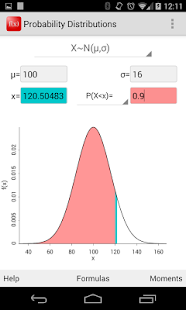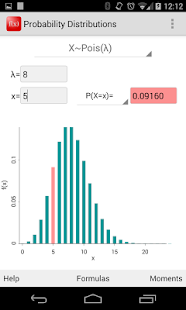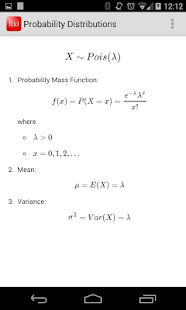# Probability Distributions

## Compute probabilities percentiles and plot various probability distributions.

4.83 EDUCATIONCompute probabilities and plot the probability mass function for the binomial, geometric, Poisson, hypergeometric, and negative binomial distributions. Compute probabilities, determine percentiles, and plot the probability density function for the normal (Gaussian), t, chi-square, F, exponential, gamma, beta, and log-normal distributions.

Some knowledge of probability distributions is required! If you don't know what a "binomial" distribution is, for example, this application will not be useful to you.

Updated SDK to version 30.

### Other versions

5.6.2 released on 20 November 2018 (1286 days ago)

### Details and Recent Ratings

Size: 0 MB
Version: 5.6.3 by Matthew Bognar
Updated: 02 November 2020 (573 days ago)
Released: 30 December 2012
Installations: more than 100 000
5 Stars: 1578
4 Stars: 104
3 Stars: 32
2 Stars: 16
1 Star: 16

### Similar apps

Android Game Categories
Android App Categories